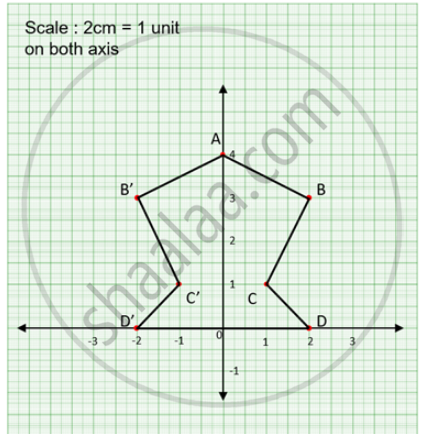# Use a Graph Paper for this Question: (Take 2cm = 1 Unit on Both X and Y Axes) (I) Plot the Following Points: A(0,4), B(2,3), C(1,1) and D(2,0). - Mathematics

Graph

Use a graph paper for this question: (Take 2cm = 1 unit on both x and y axes) (i) Plot the following points: A(0,4), B(2,3), C(1,1) and D(2,0).

(ii) Reflect points B, C, D on the y-axis and write down their coordinates. Name the images as B', C', D' respectively.

(iii) Join the points A, B, C, D, D', C', B' and A in order, so as to form a closed figure. Write down the equation to the line about which if this closed figure obtained is folded, the two parts of the figure exactly coincide.

#### Solution

(i)Plotting A(0,4), B(2,3), C(1,1) and D(2,0).(ii) Reflected points B'(-2,3), C'(-1,1) and D'(-2,0).

(iii) The figure is symmetrical about x = 0

Concept: Invariant Points.
Is there an error in this question or solution?

#### APPEARS IN

Selina Concise Maths Class 10 ICSE
Chapter 12 Reflection
Exercise 12 (B) | Q 17 | Page 171